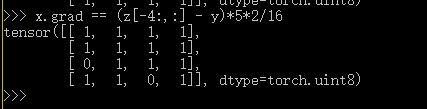# Will the slice operation on a list be traced back in autograd?

Hi there, there is a puzzle for me about whether the slice operation on a list will be traced back when autograding.
Here is a piece of script of my example

``````import torch
import torch.nn as nn
import numpy as np

criterion = nn.MSELoss()
y = torch.randn(4, 4)
z = [2*x]
for i in range(3):
z.append(z[-1]+x)

loss = criterion(z[-1], y)
loss.backward()
``````

If the slice operation were traced back when autograding, then it would be true that
`x.grad == (z[-1] - y)*5*2/16`. However, what I got wasI can not figure out what’s wrong with this situation. Any help will be appreciated.

Edit: I’m just trying to implement LISTA which is proposed by LeCun using pytorch. Maybe using RNN should be practical but I have no idea how to do it in this way.

Lists are not a pytorch thing. They have no way of storing any autograd information, and pytorch has no way of knowing that a pytorch object is in a list. (well… ‘no way’… is perhaps a bit strong, but ‘no clean way, that wouldnt have lots of broken edge-cases and be brittle and unmaintainable’ perhaps).

Well, @hughperkins thanks for your comments. Lists are not a pytorch thing, so it may be untraceable. I tried the pytorch thing `torch.cat` and the code pieces are:

``````x = torch.randn(4, 4, requires_grad=True)
y = torch.randn(4,4)
for i in range(4):
if i == 0:
z = 2*x
else:
z = torch.cat((z, z[-4:, :] + x), dim=0)

loss = critetion(z[-4:, :], y)
loss.backward()
``````

However, it is still not true that `x.grad = (z[-4:, :] - y)*5*2/16`. I got the following prints:Any comments? @richard @apaszke @SimonW Thanks a lot.

You’re seeing some floating point error @AlbertZhang

I ran your code and got the expected values:

The largest deviation is `2.3842e-07`

``````In : (x.grad - (z[-1] - y)*5*2/16).max()
You are always right, @richard. Thank you for your effort.